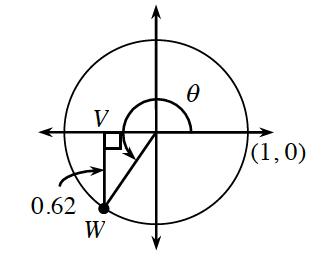### Home > PC3 > Chapter 4 > Lesson 4.2.1 > Problem4-61

4-61.Study the diagram of the unit circle at right.

If $O$ is the origin, $OW=1$. Use the Pythagorean Theorem to determine $OV$.

This angle is in Quadrant III. Should $OV$ and $VW$ each be considered positive or negative?

1. Evaluate $\sin(θ)$.

2. Evaluate $\cos(θ)$.

3. If α is another angle with the same sine as $θ$, but a different cosine, in what quadrant is $α$?

For $θ$, sine and cosine are both negative.
In what quadrant is sine negative and cosine positive?

4. If $β$ is another angle with the same cosine as $θ$, but a different sine, in what quadrant is $β$?

For $θ$, sine and cosine are both negative.
In what quadrant is sine positive and cosine negative?